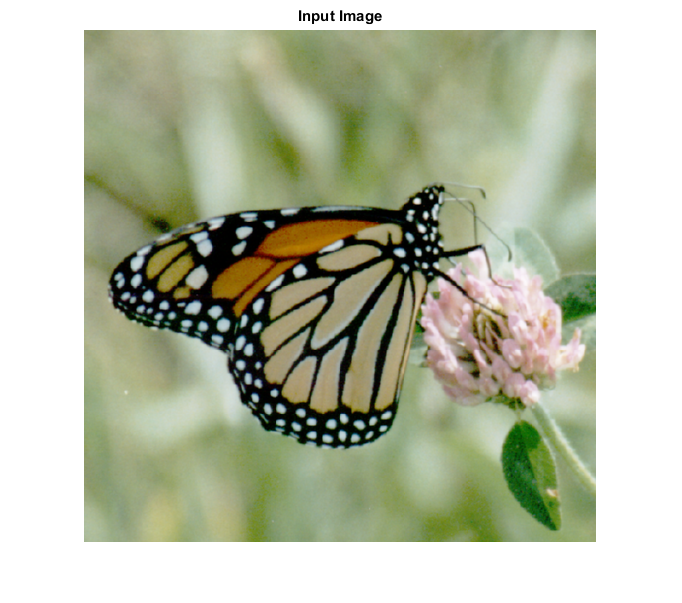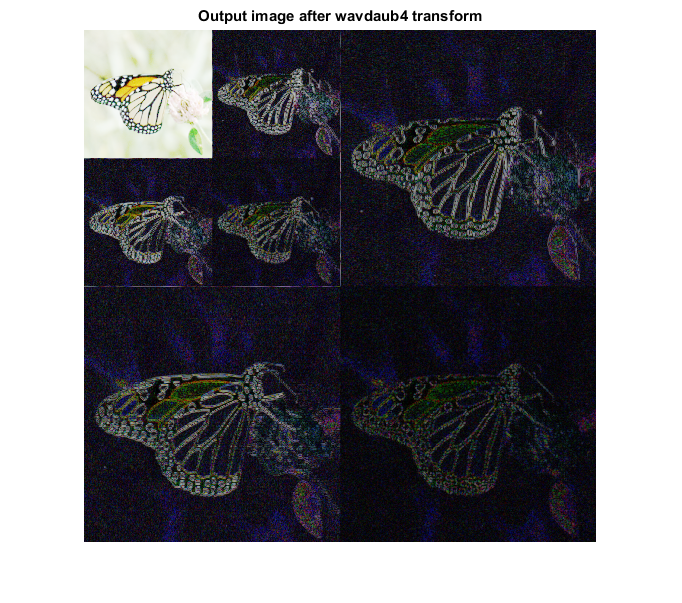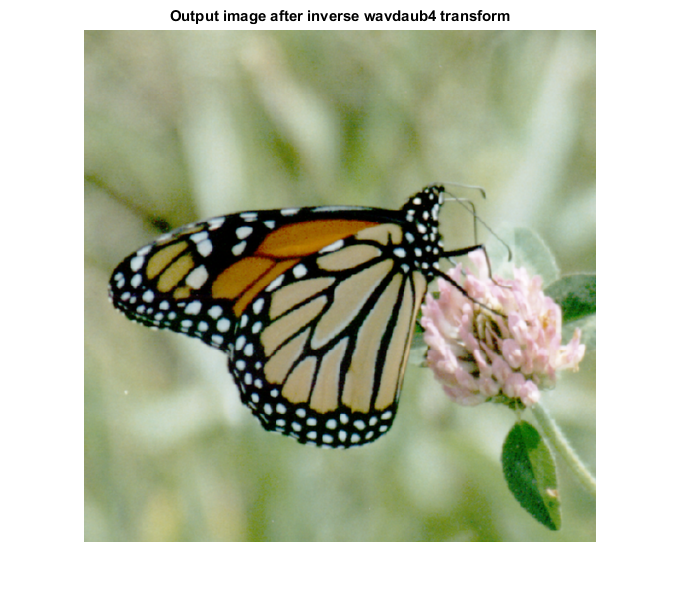# IWAVDAUB4_CVIP

iwavdaub4_cvip() - perform inverse wavelet transform based on Daubechies wavelet transform

## SYNTAX

`out_mat = iwavdaub4_cvip( img, dec )`

Input Parameters Include:

• img - The wavelet spectrum image.
• dec - The decomposition level. The same value as the forward transform must be used. An integer greater than or equal to 1.

Output Parameter Include:

• out_mat - The inverse wavelet transform of the img using Daubechies 4 wavelet coefficients. It is an image of type double.

## DESCRIPTION

This function computes the inverse wavelet transform of the input image based on the Daubechies wavelet transform. The argument block_size should match the same value given for the forward transform. For more details look at the reference below.

## REFERENCE

1. Scott E Umbaugh. DIGITAL IMAGE PROCESSING AND ANALYSIS: Applications with MATLAB and CVIPtools, 3rd Edition.

## EXAMPLE

```%Read image

% b is of type double, so it requires hist_stretch_cvip for remapping.

b = double(a);

% Decomposition level

dec = 2;

% Calling wavelet transform

w = wavdaub4_cvip(b,dec);

% This is a good way to remap

S = log(1+abs(w));

S = remap_cvip(S);

% Calling inverse transform

out_mat= iwavdaub4_cvip(w,dec);

% Display input image

figure;imshow(a);title('Input Image');

% Display Output images

figure, imshow(S,[]);title('Output image after wavdaub4 transform')  % tranforms for display.

figure; imshow(hist_stretch_cvip(out_mat,0,1,0,0),[]);title('Output image after inverse wavdaub4 transform');
```